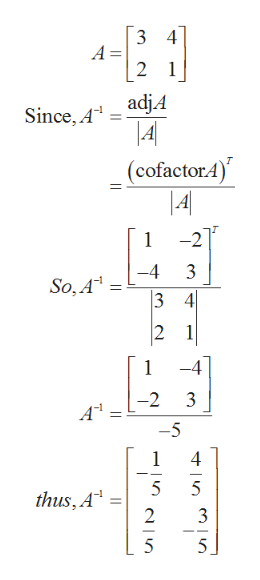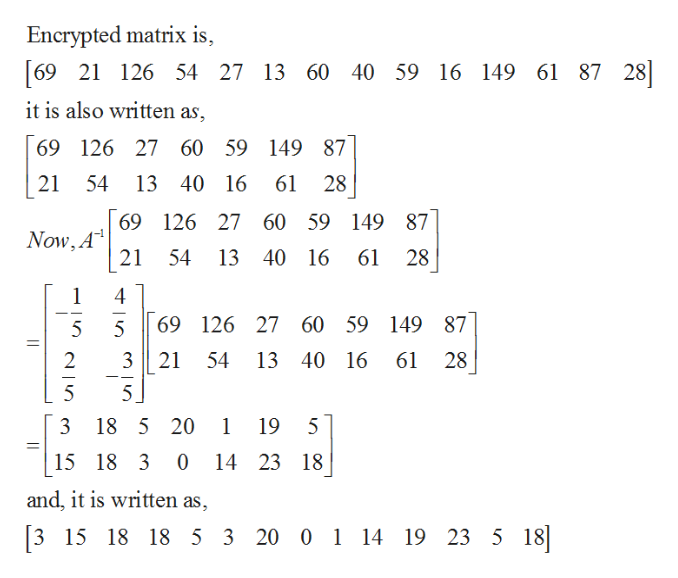# Matrices are commonly used to encrypt data. Here is a simple form such an encryption can take. First, we represent each letter in the alphabet by a number, so let us take < space > = 0, A = 1, B = 2, and so on. Thus, for example, "ABORT MISSION" becomes[1  2  15  18  20  0  13  9  19  19  9  15  14].To encrypt this coded phrase, we use an invertible matrix of any size with integer entries. For instance, let us take A to be the 2 × 2 matrix  34 21. We can first arrange the coded sequence of numbers in the form of a matrix with two rows (using zero in the last place if we have an odd number of characters) and then multiply on the left by A.Encrypted Matrix =  34 21 115201319914 2180919150  =  1111760751338742 4484035573328,which we can also write as[11  4  117  48  60  40  75  35  133  57  87  33  42  28].To decipher the encoded message, multiply the encrypted matrix by A−1. The following question uses the above matrix A for encoding and decoding.Decode the following message, which was encrypted using the matrix A. (Include any appropriate spaces in your answer.)[69  21  126  54  27  13  60  40  59  16  149  61  87  28]

Question
18 views

Matrices are commonly used to encrypt data. Here is a simple form such an encryption can take. First, we represent each letter in the alphabet by a number, so let us take < space > = 0, A = 1, B = 2, and so on. Thus, for example, "ABORT MISSION" becomes

[1  2  15  18  20  0  13  9  19  19  9  15  14].

To encrypt this coded phrase, we use an invertible matrix of any size with integer entries. For instance, let us take A to be the 2 × 2 matrix

3 4
2 1
.

We can first arrange the coded sequence of numbers in the form of a matrix with two rows (using zero in the last place if we have an odd number of characters) and then multiply on the left by A.

Encrypted Matrix  =
3 4
2 1
1 15 20 13 19 9 14
2 18 0 9 19 15 0
=
11 117 60 75 133 87 42
4 48 40 35 57 33 28
,

which we can also write as

[11  4  117  48  60  40  75  35  133  57  87  33  42  28].

To decipher the encoded message, multiply the encrypted matrix by

A−1.

The following question uses the above matrix A for encoding and decoding.

Decode the following message, which was encrypted using the matrix A. (Include any appropriate spaces in your answer.)

[69  21  126  54  27  13  60  40  59  16  149  61  87  28]
check_circle

Step 1

Consider the given question,

Find the inverse of the matrix A,help_outlineImage TranscriptioncloseA = 2 1 adj4 Since, A (cofactorA) |4 1 -4 So, A 3 4 2 1 1 -4 -2 А -5 4 5 5 thus, A 2 5 5 fullscreen
Step 2

Multiply the Encrypted matrix wit...help_outlineImage TranscriptioncloseEncrypted matrix is [69 21 28] 126 54 27 13 60 40 59 16 149 61 87 it is also written as 69 126 27 60 59 149 87 28 21 54 13 40 16 61 69 126 27 60 59 149 87 Now, A 21 54 13 40 16 61 28 1 69 126 27 60 59 149 87 5 2 3 21 54 13 40 16 61 28 5 5 18 5 20 1 19 5 0 14 23 18 15 18 3 and, it is written as 5 18] 3 15 18 18 5 3 20 0 1 14 19 23 5 |15 fullscreen

### Want to see the full answer?

See Solution

#### Want to see this answer and more?

Solutions are written by subject experts who are available 24/7. Questions are typically answered within 1 hour.*

See Solution
*Response times may vary by subject and question.
Tagged in

### Math